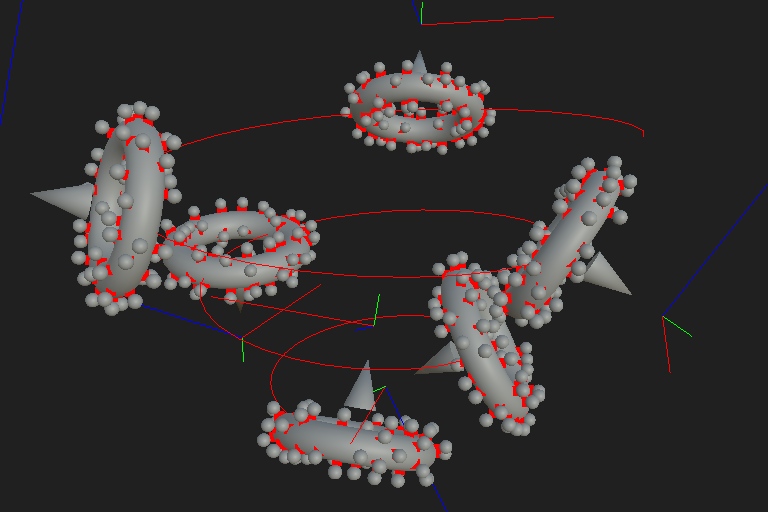# Types of Items in a scene

There are several types of Items that can coexist in a Scene: Mesh, Curve, Particle and Basis. These types can interplay to define elaborate behaviors and leverage further the power of Substance Models.

## Mesh

A Mesh is a group of Particles in 3D space (vertices) connected by segments (edges) to create surfaces (polygons).

The arrangement of vertices and how they are connected is called topology, and a mesh will react differently to specific operations depending on its topology. For instance, a Twist deformation requires a sufficient number or vertices, connected together in a way which flows with the orientation of the twist, to provide a smooth result.
Meshes can be negatively impacted by topology which compromises its integrity, such as overlapping vertices, holes, non-manifoldness, or polygons with more than 4 edges (NGons).

The way surfaces of a mesh react to light is defined by the Normal of its vertices. The normal is a vector which defines the direction the vertex/surface is facing toward, and is perpendicular to the surface of a mesh by default.
For any vertex which ‘links’ two polygons together, the there will one vertex normal defined for each polygon. If these normal are aligned, the shading from one polygon to the next will be smooth. If the normal are unaligned, the shading will be broken apart resulting in a visible shading difference between polygons.

Meshes with basic shapes can be generated using the Primitive 3D node, or you can load your own custom meshes using the Scene resource node.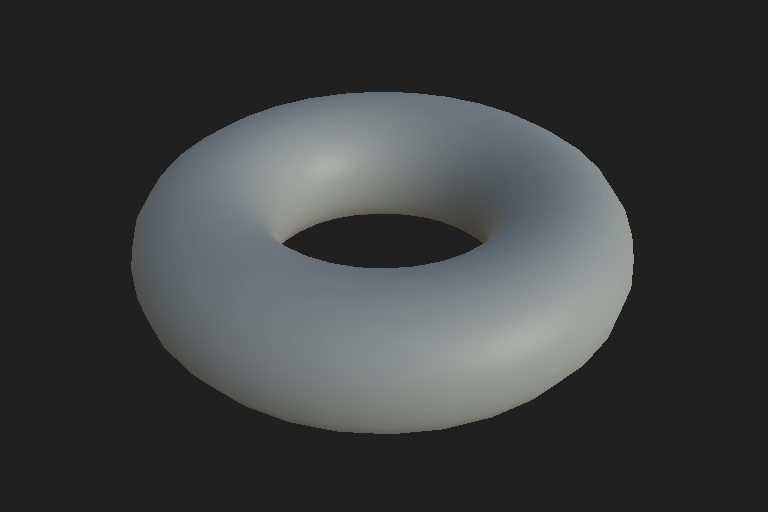### Subdivision mesh (SubD)

There is a specific type of mesh can be subdivided into a denser, smoother version dynamically: subdivision (SubD) meshes.

SubD meshes let you model geometry without the performance impact of handling very dense meshes of thousands/millions of polygons, by keeping the editable version of the mesh – i.e. the control mesh – fairly light and then subdividing it procedurally.

The control mesh is the base geometry which is used to control the shape of the subdivided/smoothed mesh. The Subdivision plane and Subdivision box generate SubD meshes and let you tweak properties of their control mesh, such as dimensions and number of faces on each axis.
In the example on the right, it is represented with a transparent surface and red vertices. The opaque mesh is the smoothed SubD result with a sharpness value of 0.

You can use a straight subdivision (i.e. infinitely sharp) which does not impact the silhouette (e.g. for sculpting purposes) or smooth out the hard angles of the mesh as it is subdivided. Because smoothing is entirely dynamic, the sharpness of the angles can be tweaked on-the-fly.

In Substance model graphs, these meshes are handled differently than standard meshes, thus meshes needs to be explicitly set as SubD meshes. This is done by using the subdivision primitives nodes mentioned above, or by setting the As Subd property of Scene resource node to True for custom meshes.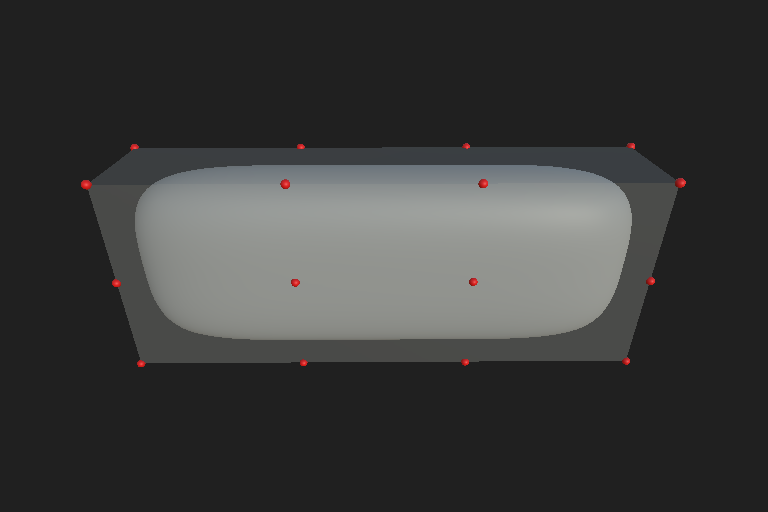## Curve

A curve is the description of a path through points in 3D space.

A curve has a specific orientation, that is, given two points A and B, A to B is different than B to A. Curves can also be closed in which case you may have a path that goes from A to B to C and back to A again.
In addition to a position, each point of a curve has a normal. The normal defines the 'twist' of the curve at a given point. All curves in Substance model graphs are Polylines, meaning that curves passed from a node to another are really lists of small segments, each of which is perfectly straight.

We use nodes such as Curve primitive, which use different interpolation styles to generate these segments, to approximate a perfectly smooth curve in space. The higher the resolution, the more segments there are, the smoother the curve looks.

The interpolation types supported at creation time are:

• Polyline is built out of multiple straight segments connected by vertices and can be subdivided into more points. This mode lets you use mathematical functions directly, such as ellipses or helixes, and each point is perfectly aligned.
• Cubic Bezier curve follows a trajectory defined by a mathematical operation. The interpolation of a curve between given 'Control points' is controlled by this math. Most of Photoshop and Illustrator curves rely internally on this type of interpolation.
• B-Spline (Basis Spline) and NURBS (Non-Uniform Rational Basis Spline) also follow trajectories defined by mathematical operations, but these curves do not pass through each point which describe them, unless multiple points overlap in the same spot. Each point has a weight which defines the magnitude of its influence on the trajectory of the curve. These curves are divided into segments of equal length for B-Splines and unequal lengths for NURBS.
Note:

Additional features and capabilities of curves are in progress.
We also plan to support these interpolations natively so their control points are still available for other nodes to use in the future. For now however, all results are baked down to Polylines.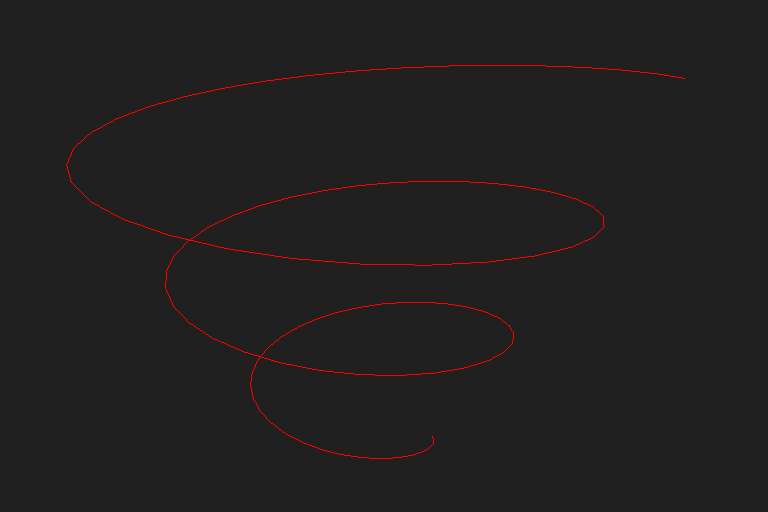## Particle

A Particle is an object representing a unique location in 3D space, with a given orientation. We commonly refer to a set of generated and scattered Particles as a Particle cloud.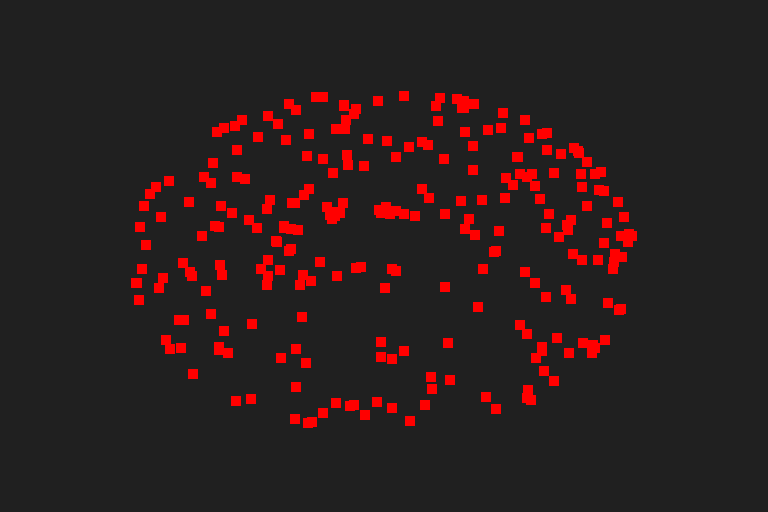## Basis

A Basis is a local coordinate space which can serve as an easier point of reference for new transformations, deformations and instancing of Elements. A Basis can adapt to the properties of other Elements, e.g. resting on top of a mesh as its height changes.

For instance, if you wish to move a mesh along the a wall, it is easier to create a new Basis aligned with that surface, and use that new reference Basis to move the mesh as if it was on the ‘floor’. If the wall changes dimensions, the Basis will follow and all Items using this Basis will move accordingly to rest on the wall.

All nodes in a Substance Model graph which take a Scene as an input will change the scene using its global world-aligned bounding box as a reference space, unless specified otherwise explicitly by a parameter. The custom Basis is one of the methods of specifying that reference space.## Interplay between item types

The Item types listed above should be used in combination to leverage the full capabilities of Substance model graphs. Here are some examples of such combinations:

• Meshes can be instantiated along a Curve
• Particles can be sampled on the surface of a Mesh to then instantiate other Meshes at their positions
• Curves can be extracted from the segments of a Mesh
• Meshes can be used to remove all Meshes they overlap with
• The transform (position, orientation, scale) of a Mesh can be used as the Basis for other Meshes or Curves
Note:
In the example on the right the Curves, Particles and Bases were displayed for demonstration purposes only. You can display these Items in the final result for checking the behaviour of your Scene, by including them in Scene merges.